# Browse & Filter

For page specific messages
For page author info
3564 records found.
Operations
Title+Summary Name Date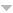### [YMP/EM-03009] Electrostatic Energy of a Parallel Plate Condenser

Node id: 6028page
23-12-04 23:12:46 n

### [YMP/EM-03006] Potential of a solid sphere

Node id: 6027page
23-12-04 23:12:16 n

### [YMP/EM-02010] Electric Field of Overlapping Uniformly Charged Spheres

Node id: 6023page
 Problem Two spheres, each of radius $R$ and carrying charge densities $+\rho$ and $-\rho$ respectively, are placed so that they partially overlap. The separation between the centers of the spheres is $D$. Show that the field in the region of overlap is constant and find its value.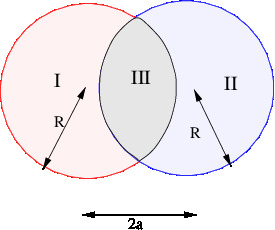23-12-04 05:12:08 n

### [LECS/EM-03002]-Examples of Computation of Electric Potential

Node id: 5652page

We give details of computation of potential for the following examples.
1. Uniformly Charged Ring
2. Uniformly Charged Disc
3. Thin Spherical Shell
4. Uniformly Charged Solid Sphere

23-11-28 16:11:52 n

### [LECS/EM-03003]-Electrostatic Energy

Node id: 5653page

The electrostatic  energy of a continuous charge distribution is defined as the energy required to assemble the charges at  infinity into the positions as in the given distribution. For a continuous charge distribution it is shown to be $$\dfrac{\epsilon_0}{2}\iiint(\vec E\cdot\vec E) dV$$ . Thus a volume of space having nonvanishing electric field has energy density  $$\dfrac{\epsilon_0}{2}(\vec E\cdot\vec E)$$.The expression for the electrostatic energy reduces to the usual answer $$\frac{1}{2} CV^2$$ for a charged parallel plate capacitor. For a  uniformly charged sphere of radius $$R$$ the electrostatic energy is proved to be equal to $$\frac{3}{5}\Big(\frac{Q^2}{4\pi\epsilon_0 R^2} \Big)$$.

23-11-28 16:11:21 n

### [LECS/EM-03001]-Electric Potential

Node id: 5651page

The concept of electric potential for static electric field is defined as work done on a unit charge. The expression for the electric potential of a  $$q$$ charge is obtained. For a system of point charges  the potential can be written down as superposition of potential due to individual charges. As an illustration we compute potential due to a dipole.

23-11-28 15:11:04 n

### [LECS/EM-03004]-Multipole Expansion

Node id: 5654page

Large distance expansion of electric potential of a charge distribution in a finite voluem $$V$$ is presented. Expressions for dipole and quadrupole moments are obtained.

23-11-28 15:11:03 n

### [LECS/EM-03005]-Maxwell’s Equations for Electrostatics

Node id: 5655page

The Maxwell's equations for electrostatic are derived from Coulomb's law which has been formulated based on experiments. This provides initial experimental evidence for the Maxwell's equations. We discuss two applications of Maxwell's equations. The first result is that  the electric field inside an empty cavity in conductors is proved to be zero. The second result is  an  expression for electric stress tensor is derived. The surface integral of the electric tensor gives the force on charge distribution.

23-11-28 15:11:01 n

### [LECS/EM-02003] Gauss Law

Node id: 6025page

The flux of electric field through a surface in defined as a surface integral and the statement of Gauss law is given. A few examples of computing the electric field using Gauss law and symmetry of the problem are discussed. A simple and intuitive proof of Gauss law is given following Feynman lectures. The task of proving Gauss law for arbitrary charge distribution is reduced to the problem proving the Gauss law for a single point charge by appealing to the superposition principle.

23-11-27 12:11:56 n

### [LECS/EM-02001] Coulomb's Law and Electric Field

Node id: 6012article

The Coulomb's law gives the force between two point charges. It can be reformulated as giving electric field of a point charge which in turn gives rise to force on a nearby point charge.The electric field of a point charge is generalized to system with continuous charge distributions. Expression for the electric field of  line surface and volume charge distiibutions are given. The examples of computation of electric field of (a) Line charge, (b) Uniformly charged loop,  (c) Thin Spherical Shell and (d)  Dipole are discussed.

23-11-27 11:11:16 n

### [YMP/EM-All] Solved Problems in Electromagnetic Theory

Node id: 5918collection

Work Under Progress

(LUPDate : Nov 24, 2023)

23-11-26 18:11:08 n

### [Feyn-II-Sec14]

Node id: 6026page
23-11-24 10:11:27 n

### [YMP/EM-02012] Average Value of Electric Field

Node id: 6021page

Problem

A solid sphere of radius $$R$$ carries a charge density $$\rho(\vec{r})$$. Show that the average of the electric field inside the sphere is $\vec{E}= - \frac{1}{4\pi\epsilon_0} \frac{\vec{p}}{R^3},$ where $$\vec{p}$$ is the total dipole moment of the sphere.

23-11-23 22:11:27 n

### [YMP/EM-02011] Overlapping Spheres -II

Node id: 6024article
 ProblemTwo spheres each of radius $R$ are placed so that they partially overlap. The charge densities in the overlap region is zero and in the two non overlapping regions is $+\rho$ and $-\rho$ respectively as shown in figure. The separation between the centers of the spheres is $D$.Find the electric field in the two non-overlap regions.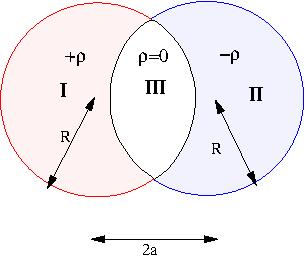23-11-23 22:11:38 n

### [YMP/EM-02009] Electric Field of a Uniformly Charged Sphere

Node id: 6022page
 Show that the electric field of a uniformly charged sphere, radius $$R$$, total charge $$Q$$ has the magnitude given by$E= \begin{cases} E_0 x & x< 1 \\ E_0/x^2 & x>1 \end{cases}$where $$E_0= \dfrac{Q}{4\pi\epsilon_0 R^2 }$$   and $$x=\dfrac{r}{R}$$.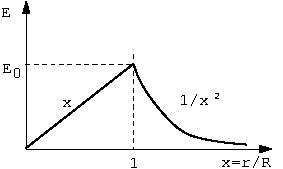23-11-23 22:11:47 n

### [YMP/EM-02008] Electric Field Due to a Dipole

Node id: 6013page

A dipole is a system of two equal and opposite charges separated by a distance. Its electric field is computed and the field at large distances depends only on the dipole moment, defined as the product of the charge and separation between the charges.

While dipole is a system of two charges, the concept of dipole moment is defined and is useful for any system of charges  with total zero charge.

23-11-23 22:11:57 n

### [YMP/EM-02007] Electric Field of a Uniformly Charged Spherical Shell

Node id: 6020page
23-11-23 22:11:50 n

### [YMP/EM-02006] Electric Field due to a Uniformly Charged Disk

Node id: 6019page

We compute the electric field  due to a uniformly charged disk at a point on the axis of the disk.

23-11-23 22:11:28 n

### [YMP/EM-02004] Electric Field due to a Line with Uniform Charge Density

Node id: 6016page

Let $$AB$$ be a line charge with charge per unit length $\lambda$, which is given to be uniform. The field is to be computed at a point $$P$$,. Let $$p$$ be the perpendicular distance of $$P$$ from the line $$AB$$. let $$\alpha, \beta$$ be the angles as shown in the figure. Electric field will be calculated at a general point $$P$$, the corner point $$C$$ and the middle point $$M$$ as shown in figure below.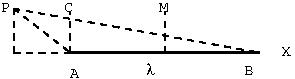23-11-23 22:11:35 n

### [YMP/EM-02003] Dipole Moment of Overlapping Spheres

Node id: 6015page
 Two spheres each of radius $R$ are placed so that they partially overlap. The charge densities in the overlap region is zero. Outside the overlap region but inside the spheres the charge densities in the two non overlapping   regions are $+\rho$ and $-\rho$, respectively, as shown in figure. The charge density in the overlap region will be zero. The separation between the centers of the spheres is $D$.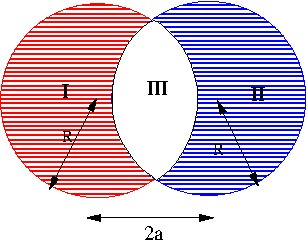1. Guess the value of the dipole moment?
2. Compute the dipole moment of the system and check the correctness of the expression you guessed.
3. How will the dipole moment change if the two spheres equal and opposite total charges but have different radii $$a,b, a \ne b$$.
23-11-23 22:11:53 n

X